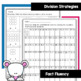# Bilingual Division Word Problems in English & SpanishSubject
Resource Type
File Type

PDF

(8 MB|37 pages)
Product Rating
4.0
(20 Ratings)
Standards
Also included in:
1. Bilingual Multiplication and Division Word Problems Bundle are two products that focus on word problems and computation. Each worksheet is in English and Spanish so that all of your English Language Learners can work at the same time. This bundle was created as a resource that you can use in your B
\$9.99
\$8.00
Save \$1.99
2. Bilingual Math Mega Bundle contains 6 different math concepts. Each product contains worksheets that are both in English and Spanish. With these all of your English Language Learners can work together within the same lesson.
\$39.97
\$36.00
Save \$3.97
3. SAVE \$\$ by purchasing 4 Math Units for a discounted price. The Third Grade Bilingual Math bundle are products meant to compliment your Bilingual or ESL curriculum. Each worksheet has an identical worksheet in English and Spanish. Meet all of your English Language Learners needs by having these wor
\$38.99
\$38.00
Save \$0.99
4. Everything you need to teach the Third Grade Math Common Core curriculum are included in this bundle. Save \$\$\$ by purchasing all 18 products for one low price. Included are no - prep worksheets to teach multiplication, division, fractions, rounding, and addition with regrouping. Also included are
\$139.94
\$100.00
Save \$39.94
5. The Multiplication Word Problems no prep worksheets are a great introduction to an essential third and fourth grade mathematics skill. Once my students have mastered understanding Multiplication word problems, I introduce the Division word problems worksheets followed by the Multiplication and Divi
\$15.99
\$12.00
Save \$3.99
6. Everything you need to teach the Third Grade Math Common Core curriculum are included in this bundle. Save \$\$\$ by purchasing all 19 products for one low price. Included are no - prep worksheets to teach multiplication, division, fractions, rounding, and addition with regrouping. Also included are
\$129.94
\$100.00
Save \$29.94
7. The Multiplication Word Problems no prep worksheets are a great introduction to an essential third and fourth grade mathematics skill. Once my students have mastered understanding Multiplication word problems, I introduce the Division word problems worksheets followed by the Multiplication and Divi
\$15.99
\$12.00
Save \$3.99
• Product Description
• StandardsNEW

Do your English Language Learners need help with division word problems? This fabulous variety of Spanish and English division word problems can help. No need to translate word problems because these bilingual no prep worksheets do the work for you.

Fluently multiply and divide within 100, using strategies such as the relationship between multiplication and division (e.g., knowing that 8 × 5 = 40, one knows 40 ÷ 5 = 8) or properties of operations. By the end of Grade 3, know from memory all products of two one-digit numbers.
Apply properties of operations as strategies to multiply and divide. Examples: If 6 × 4 = 24 is known, then 4 × 6 = 24 is also known. (Commutative property of multiplication.) 3 × 5 × 2 can be found by 3 × 5 = 15, then 15 × 2 = 30, or by 5 × 2 = 10, then 3 × 10 = 30. (Associative property of multiplication.) Knowing that 8 × 5 = 40 and 8 × 2 = 16, one can find 8 × 7 as 8 × (5 + 2) = (8 × 5) + (8 × 2) = 40 + 16 = 56. (Distributive property.)
Determine the unknown whole number in a multiplication or division equation relating three whole numbers. For example, determine the unknown number that makes the equation true in each of the equations 8 × ? = 48, 5 = __ ÷ 3, 6 × 6 = ?.
Use multiplication and division within 100 to solve word problems in situations involving equal groups, arrays, and measurement quantities, e.g., by using drawings and equations with a symbol for the unknown number to represent the problem.
Total Pages
37 pages
N/A
Teaching Duration
N/A
Report this Resource to TpT
Reported resources will be reviewed by our team. Report this resource to let us know if this resource violates TpT’s content guidelines.